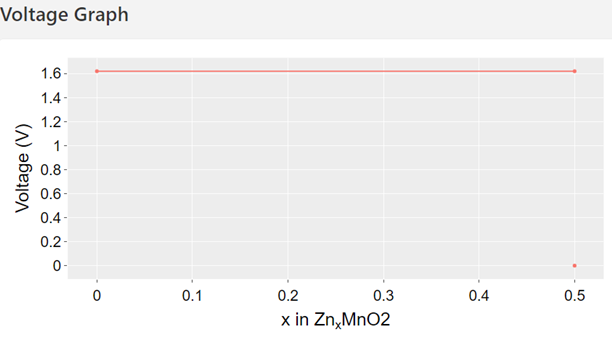# Chemical Potential Sources from Phase Diagram

Hello,

I have calculated chemical potentials for a 3 component phase diagram using pymatgen. However, I do not fully understand how chemical potential is calculated. Are temperature corrections taken into account (especially for gas phase)? Is there an attribute I can use to see which structure is being used to calculate the chemical potential?

Please see my code attached and a snippet of the output below.
Sample Code

``````from pymatgen.core.composition import Composition
from pymatgen.core.periodic_table import Element
from pymatgen.ext.matproj import MPRester
from pymatgen.analysis.phase_diagram import PhaseDiagram

api_key = None

#Get data for 3 component system
with MPRester(api_key) as m:
entries = m.get_entries_in_chemsys(['Fe', 'Ti', 'O'])

#Generate phase diagram
pd = PhaseDiagram(entries)

#Generate reaction pathways
element_profile = pd.get_element_profile(element=Element('O'), comp=Composition('FeTiO3'))

#Print relevant data
for i, evolution_step in enumerate(element_profile):
print('Reaction: {}'.format(evolution_step['reaction']))
print('Chemical Potential: {}'.format(evolution_step['chempot']))
print('Reaction object fields: {}'.format(evolution_step['reaction'].__dict__.keys()))
print('Components of chemical reaction data:')
for comp in evolution_step['reaction']._all_comp:
print('\t{}'.format(comp.__dict__))
print('-')
``````

Snippet of Output
Reaction: TiFeO3 + 0.25 O2 -> TiO2 + 0.5 Fe2O3
Chemical Potential: -4.93552791875
Reaction object fields: dict_keys([’_input_reactants’, ‘_input_products’, ‘_all_comp’, ‘_els’, ‘_coeffs’])
Components of chemical reaction data:
{‘allow_negative’: False, ‘_natoms’: 5.0, ‘_data’: {Element Fe: 1.0, Element Ti: 1.0, Element O: 3.0}}
{‘allow_negative’: False, ‘_natoms’: 12.0, ‘_data’: {Element Ti: 4.0, Element O: 8.0}}
{‘allow_negative’: False, ‘_natoms’: 40.0, ‘_data’: {Element Fe: 16.0, Element O: 24.0}}
{‘allow_negative’: False, ‘_natoms’: 1.0, ‘_data’: {Element O: 1.0}}
-

Any help would be greatly appreciated.
Jon

I’ll need to double check this, but I believe the chemical potentials printed here are the energy per atom that would be assigned to the O2 species (treated as an independent variable in context with the other entry energies as determined from our simulations). Thus, there isn’t a corresponding structure per se.

If you want a more explicit reference, you can use the energy per atom of the most stable oxygen energy in the MP database (mp-12957, -4.9355 eV/atom). Because of MP’s correction scheme, this value roughly corresponds to a reference chemical potential of O2 gas at STP. You could then estimate the change in chemical potential as a function of T and P using some model (e. g. an equation of state).

Ah, I see. Thank you for the help!

Hi all,

I have a question related to the reference of chemical potential of O2. I tried to replicate the O2 evolution diagram for FePO4 in the battery explorer on the Materials Project website. I can reproduce different ‘steps’ of O2 evolution with the right decomposition reactions but couldn’t figure out how to get the same values of muO2. Here is the code I used:

Code snippet

from pymatgen import MPRester, Composition, Element
from pymatgen.io.vasp import Vasprun
from pymatgen.analysis.phase_diagram import *
from pymatgen.entries.computed_entries import ComputedEntry
from pymatgen.core.composition import Composition
from pymatgen.entries.compatibility import MaterialsProjectCompatibility

ref_element = ‘O’
mp_id = ‘mp-540111’

api_key = None

rester = MPRester(api_key)
entry = rester.get_entry_by_material_id(mp_id)
entries = rester.get_entries_in_chemsys([‘Fe’, “P”, “O”])

pd = PhaseDiagram(entries)

ref_entries = [e for e in entries if e.composition.reduced_formula == ‘O2’]
u_ref_0 = min(ref_entries, key=lambda e: e.energy_per_atom).energy_per_atom
print(u_ref_0)

el_profile = pd.get_element_profile(Element(ref_element), entry.composition)
for i, d in enumerate(el_profile):
voltage = d[“chempot”] - u_ref_0
print(“Voltage: 0.5s eV" voltage)
print(d[“reaction”])
print(”")

Here is what I got:
Voltage: 0.0 eV
4 FePO4 -> 4 FePO4

Voltage: -1.85 eV
4 FePO4 -> 0.4 Fe7(PO4)6 + 0.4 Fe3(P2O7)2 + 0.4 O2

Voltage: -1.98 eV
4 FePO4 -> 0.6667 Fe3(P2O7)2 + 0.6667 Fe3(PO4)2 + 0.6667 O2

Voltage: -2.26 eV
4 FePO4 -> 2 Fe2P2O7 + O2

Voltage: -3.52 eV
4 FePO4 -> 4 FeP + 8 O2

While on Material Project, they got this:

Any help would be greatly appreciate.

Best
Seiha.

Hi SSeiha,
The battery database is currently out of date so some of the materials in the muO2 plot are missing on the website. If you generated your own muO2 plot and it looks qualitatively similar then I wouldn’t worry about it.
Best,
-Jimmy

1 Like

Dear Mr.jshen,

I have a question about Voltage graph,
After DFT calculation, how can I use Pymatgen code for plot the Voltage Graph like Matrial Project as bellow?

Can you introduct some code for make this plot?
Thank You so much for helping?

There is a plotter module here:

Dear Mr.jshen,
Because I am a beginer for Pymatgen, so is this code for jupyter notebook or for other environments?
I usually use code samilar with codes in the bellow picture:

So how can I use the code at bellow for batteries in jupyter notebook?
" materialsproject/pymatgen/blob/6dacfff5e7e81a32b16153ddbfb7c31ebfcb83d0/pymatgen/apps/battery/plotter.py "
Thank you so much for helping!

Hi @buithitham,
Yes, you can import the pyton code from a jupyter notebook since directly just import the module in jupyter:

So all of the code is python code, Jupyter is only one easy way to interact with python code.

Hello, I am running the code as bellow for calcualtion voltage for ZnMn2O4 cathode material,

``````from mp_api.client import MPRester
from pymatgen.entries.computed_entries import ComputedEntry
from pymatgen.analysis.phase_diagram import (CompoundPhaseDiagram,PDPlotter,PhaseDiagram,)
from pymatgen.analysis.interface_reactions import GrandPotentialInterfacialReactivity, InterfacialReactivity
from pymatgen.core import Composition, Element
API=‘API KEy'
rester = MPRester(API)
mp_id = 'mp-18751’
entry = rester.get_entry_by_material_id(mp_id)
mp_entries = rester.get_entries_in_chemsys(["Zn", "Mn", "O"])
compatibility = MaterialsProjectCompatibility()
entry = compatibility.process_entry(entry)
entries = compatibility.process_entries([entry] + mp_entries)
pd = PhaseDiagram(entries)

comp = Composition("ZnMn2O4")
ref_entries = [e for e in entries if e.composition.reduced_formula == "Zn"]
u_ref_0 = min(ref_entries, key=lambda e: e.energy_per_atom).energy_per_atom
print(u_ref_0)
el_profile = pd.get_element_profile(element=Element("Zn"), comp=comp)

for i, d in enumerate(el_profile):
voltage = -(d["chempot"] - u_ref_0)/2
print("Voltage: %s V" % voltage)
print(d["reaction"])
print("")
``````

Final I have the results :
Voltage: -0.0 V
Mn2ZnO4 + Zn → 2 ZnO + 2 MnO

Voltage: 0.5521237599999981 V
Mn2ZnO4 + 0.3333 Zn → 0.6667 Mn3O4 + 1.333 ZnO

Voltage: 0.7020465324999853 V
Mn2ZnO4 → Mn2ZnO4

Voltage: 1.520648380000003 V
Mn2ZnO4 → 2 MnO2 + Zn
However, when I am check the voltage graph of ZnMn2O4 (mp-18751) in Material Projects
, it show different value ( 1.619 V):So can you let me know what is problem in my calculation?
Thank you so much for helping!